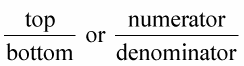Fractions just have two parts: a numerator and a denominator. The denominator, or bottom number, indicates the total number of items. The numerator, or top number, tells you how many of the total (the bottom number) are being considered.You can remember the exact placement of the numbers and their proper names if you think in terms of direction:

• The numerator is always north (on top of) the fraction line.

• The denominator is always down below the fraction line.

In all the cases using fractions, the denominator tells you how many equal portions or pieces there are. Without the equal rule, you could get different pieces in various sizes. For instance, in a recipe calling for 1/2 cup of flour, if you didn’t know that the one part was one of two equal parts, then there could be two unequal parts — one big and one little.

Check out these examples of common fractions to understand how fractions work:

3/4 = 3 out of 4 equal parts

1/2 = 1 out of 2 equal parts

2/3 = 2 out of 3 equal parts

Understanding fractions, where they come from, and why they look the way they do helps when you’re solving problems that contain fractions.